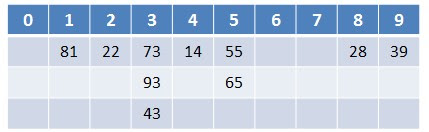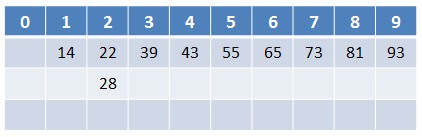## 2011年10月26日 星期三

### [ Algorithm in Java ] 排序 : 基數排序法

73, 22, 93, 43, 55, 14, 28, 65, 39, 8181, 22, 73, 93, 43, 14, 55, 65, 28, 3914, 22, 28, 39, 43, 55, 65, 73, 81, 93

1. package alg.sort;
2.
4.     private static int[][] bucks;
5.     private static int[] index = new int;
6.
7.     /**
8.      * BD : Sort number with radix sort algorithm.
9.      * @param number
10.      *      The matrix to be sorted
11.      * @param n
12.      *      The max figure of all elements in matrix number.
13.      */
14.     public static void sort(int[] number, int n)
15.     {
16.         bucks = new int[number.length];
17.
18.         int rdx = 0;
19.         int rst = 0;
20.         for(int i=0; i
21.         {
22.             /*Put into bucks*/
23.             for(int j=0; j
24.             {
25.                 if(i==0) rst = number[j]%10;
26.                 else
27.                 {
28.                     rdx = (int)Math.pow(10, i);
29.                     rst = (number[j]/rdx)%10;
30.                 }
31.                 bucks[rst][index[rst]++] = number[j];
32.             }
33.             /*Restore from bucks*/
34.             int p=0;
35.             for(int j=0; j<10; j++)
36.             {
37.                 for(int k=0; k
38.                 {
39.                     System.out.printf("\t[Restore from bucks] number[%d]=%d...\n", p, bucks[j][k]);
40.                     number[p++]=bucks[j][k];
41.                 }
42.                 index[j]=0;
43.             }
44.             System.out.println();
45.         }
46.     }
47.
48.     public static void main(String args[])
49.     {
50.         int numbers[] = {73229343551428653981};
52.         for(int i=0; i" ");
53.     }
54. }

[ Data Structures with Java ] Section 15.2 : The Radix Sort
The method, called the radix sort, is a linear algorithm that uses successive digits in the elements to partially sort the array. The technique is two-stage process refereed to as transform-and-conquer...
This message was edited 6 times. Last update was at 27/10/2011 10:27:31

### [NodeJS 文章收集] NodeJS accessing file with relative path

Source From  Here Question It seemed like a straight forward problem. But I am not able to crack this. Within  helper1.js  I would like to a...